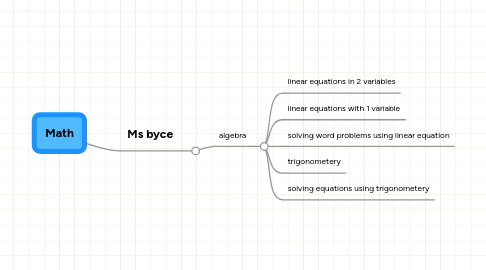# Math

Get Started. It's FreeMath by## 1. Ms byce

### 1.1. algebra

1.1.1. linear equations in 2 variables

1.1.2. linear equations with 1 variable

1.1.3. solving word problems using linear equation

1.1.4. trigonometery

1.1.5. solving equations using trigonometery